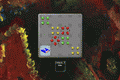-=+=- -=+=- -=+=- -=+=- -=+=- -=+=- -=+=- -=+=- -=+=- -=+=- -=+=- -=+=- -=+=- -=+=- -=+=- -=+=- -=+=- -=+=- -=+=- -=+=- -=+=- -=+=- -=+=- -=+=- -=+=- -=+=- -=+=- -=+=- -=+=- -=+=- (c) WidthPadding Industries 1987 0|60|0 -=+=- -=+=- -=+=- -=+=- -=+=- -=+=- -=+=- -=+=- -=+=- -=+=- -=+=- -=+=- -=+=- -=+=- -=+=- -=+=- -=+=- -=+=- -=+=- -=+=- -=+=- -=+=- -=+=- -=+=- -=+=- -=+=- -=+=- -=+=- -=+=- -=+=-
SoCoder -> Showcase Home -> Puzzle

PioCreated : 11 January 2008
Edited : 15 December 2008
System : Nintendo DS
Language : FastBasic

### Poca

remove all arrows

game site
ScreenshotsOn 4x4 field, level after level, you remove the arrows in certain order.
This game features many levels, occasional music and rudimentary background story.
It is my first game, so code is a mess.

Friday, 11 January 2008, 23:15
blanko1324When I first started playing, I didn't know what the hell was going on; even after reading the instructions!

But I got it eventually, and I must admit, you've got a really cool concept here. I got to Street 5 before my brain just died out. It sent me back to Street 4 and I just didn't want to go through that one again; it was a tough one. Plus, it's one in the morning.

Next version could use a timer element, to make things more challenging. Really nice work so far. I had fun. I'll play some more soon.Saturday, 12 January 2008, 00:57
HoboBenOh wow I just played the online version; very cool indeed!
Saturday, 12 January 2008, 10:06
PioThanks!

Yes, the timer... some elements could change over time, and parts of a level would have to be done quickly, or in coordination with other parts. That would be more exciting, but not much more challenging as it still depends on level design. Maybe if I put a level editor (for that hypothetical new game) online, so anybody interested could make and test levels? ...Saturday, 12 January 2008, 18:09
power mouseyhey Pio,

this looks fun.do the Poca....cheers
Sunday, 13 January 2008, 03:53
PhoenixI can't download the game -- I don't get a message saying it's a 404 error but it looks like one. Perhaps the server is just down at the moment.
Sunday, 13 January 2008, 04:45
Jayenkai